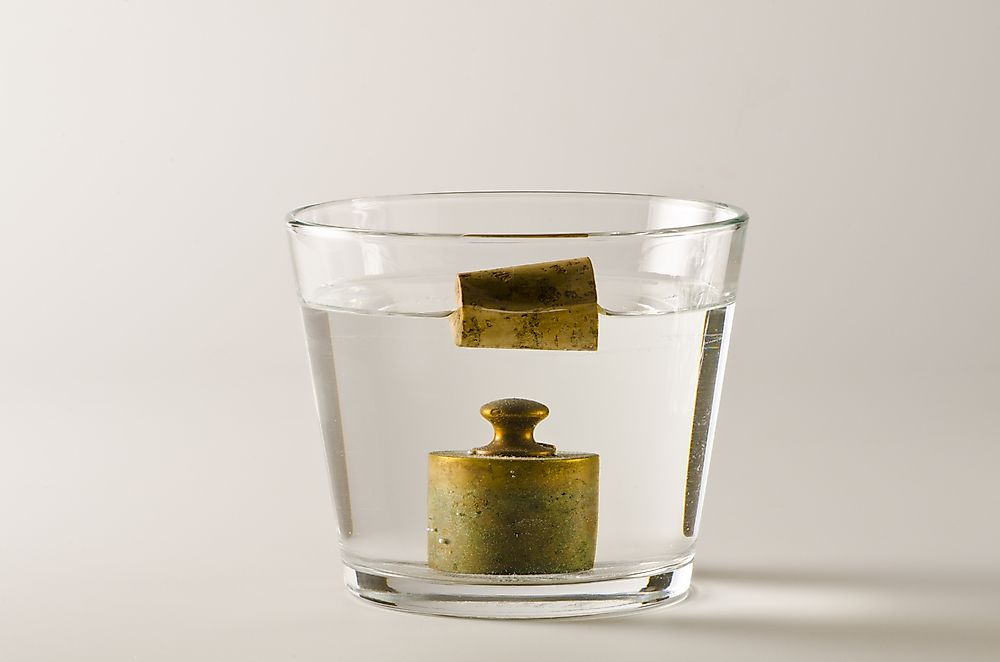# What is Density?

By Mercie Chelaa on January 10 2018 in Did You KnowObjects will float or sink based on their density in comparison to the density of water.

Just like any other units of measurement, density has a variety of uses and applications in various fields. It has been used by scientists to solve problems, especially geological ones.

## What Is Density?

Density is a unit used to measure the compactness of a substance, and it is mostly calculated in kilograms per cubic meter (Kg/m3) or grams per cubic centimeter (g/cm3). Therefore, the density of every object can be averagely equated with the summation of its mass divided by the aggregated volume of the object. In simple terms, density is the number of kilograms that a one-meter-cubed object weighs.

Every substance has its own density since each is made of different molecules and atoms. Therefore, it is not the bulk of an object that determines the density but the mass. For example, the metal, wood, and plastic of the same size have different densities because they have different masses. They have different masses because each differs in the way atoms are packed to make up the object. For example, metals like copper, iron, and lead have more closely packed atoms compared to plastic and wood, therefore the higher masses of these metals is what makes them denser than the wood and plastic.

It is also important to consider the fact that mass is not weight and that mass remains constant despite changes in place. However, weight changes. For example, an individual's body weight on Earth will change if the individual travels to the Moon.

## How to Calculate Density

To make it easier to understand, we will look an example of how to calculate the density of a substance. Firstly, you may have to recall that density is the volume divides by the mass of the same object. We will start by looking at a cubed-object with each side measuring 4 meters with a mass of 5 kilograms.

Now that we need both volume and mass, we will first calculate the volume whereby we will multiply the length (L), width (W), and height (H); hence, Volume (V)=4m*4m*4m, giving us 64 cubic meters. And since mass is 5 kilograms, then density=volume divide by mass., which is 64 cubic meters divides by 5 kilograms. Thus, the density of the above object is 12.8kg/m3.

## Application of Density

Application of density is wide. It ranges from shipbuilding to identification of rocks. To get an in-depth understanding, let us briefly discuss how it is applied to floating objects in the water.

Some objects sink or rise depending if they are gases or liquids. The determinant whether these objects sink or rise is the density of the liquids or gases on which they are suspended. Therefore, understanding the concept of density help in knowing why objects sink or float in water and the motions of objects and gases in the atmosphere. An example is a ship that floats in the water despite its weight and size. You may say that the ship is made of steel which is a metal with higher density than water; the ship is built in a manner that its volume is large, hence when the ship mass is divided with its volume, it comes less than 1.0g/cm3 which is the density of the water. The ship must remain with a lesser density than water even when loaded or it will sink.

Density is also used for mineral identification and comprehending rock composition. It also controls ocean currents and circulation.